# 14.15: Organic Reactions

$$\newcommand{\vecs}{\overset { \rightharpoonup} {\mathbf{#1}} }$$ $$\newcommand{\vecd}{\overset{-\!-\!\rightharpoonup}{\vphantom{a}\smash {#1}}}$$$$\newcommand{\id}{\mathrm{id}}$$ $$\newcommand{\Span}{\mathrm{span}}$$ $$\newcommand{\kernel}{\mathrm{null}\,}$$ $$\newcommand{\range}{\mathrm{range}\,}$$ $$\newcommand{\RealPart}{\mathrm{Re}}$$ $$\newcommand{\ImaginaryPart}{\mathrm{Im}}$$ $$\newcommand{\Argument}{\mathrm{Arg}}$$ $$\newcommand{\norm}{\| #1 \|}$$ $$\newcommand{\inner}{\langle #1, #2 \rangle}$$ $$\newcommand{\Span}{\mathrm{span}}$$ $$\newcommand{\id}{\mathrm{id}}$$ $$\newcommand{\Span}{\mathrm{span}}$$ $$\newcommand{\kernel}{\mathrm{null}\,}$$ $$\newcommand{\range}{\mathrm{range}\,}$$ $$\newcommand{\RealPart}{\mathrm{Re}}$$ $$\newcommand{\ImaginaryPart}{\mathrm{Im}}$$ $$\newcommand{\Argument}{\mathrm{Arg}}$$ $$\newcommand{\norm}{\| #1 \|}$$ $$\newcommand{\inner}{\langle #1, #2 \rangle}$$ $$\newcommand{\Span}{\mathrm{span}}$$$$\newcommand{\AA}{\unicode[.8,0]{x212B}}$$

Learning Outcomes

• Identify and describe substitution, elimination, addition, hydrolysis, and condensation reactions.
• Predict products of each reaction type.
• Predict products of hydration reactions based on Markovnikov's rule.
• Explain why condensation reactions are also called dehydration reactions.
• Define "polymer".

Organic reactions require the breaking of strong covalent bonds, which takes a considerable input of energy. In order for relatively stable organic molecules to react at a reasonable rate, they often must be modified with the use of highly reactive materials or in the presence of a catalyst. In this lesson, you will learn about several general categories of organic reactions.

## Substitution Reactions

A substitution reaction, which is the same as a single replacement reaction in inorganic reactions, is a reaction in which one or more atoms in a molecule are replaced with another atom or group of atoms. Alkyl halides are formed by the substitution of a halogen atom for a hydrogen atom. When methane reacts with chlorine gas, ultraviolet light can act as a catalyst for the reaction.

$\ce{CH_4} \left( g \right) + \ce{Cl_2} \left( g \right) \overset{\text{UV light}}{\rightarrow} \ce{CH_3Cl} \left( g \right) + \ce{HCl} \left( g \right)$

The reaction produces chloromethane and hydrogen chloride. When the mixture is allowed to react for longer periods of time, further substitution reactions may occur.

$\ce{CH_3Cl} \left( g \right) + \ce{Cl_2} \left( g \right) \overset{\text{UV light}}{\rightarrow} \ce{CH_2Cl_2} \left( g \right) + \ce{HCl} \left( g \right)$

The product above is dichloromethane. Further substitution products trichloromethane and tetrachloromethane, commonly called carbon tetrachloride. A mixture of products occurs in the reaction, with the relative amounts dependent upon the time that the reaction is allowed to proceed. Chlorofluorocarbons are produced by reacting chloroalkanes with $$\ce{HF}$$, because the fluorine atom makes a stronger bond to the carbon atom than chlorine does.

$\ce{CCl_4} \left( g \right) + \ce{HF} \left( g \right) \overset{\ce{SbF_5}}{\rightarrow} \ce{CCl_3F} \left( g \right) + \ce{HCl} \left( g \right)$

The fluorine atom substitutes for a chlorine atom in the reaction.

## Elimination Reactions

An elimination reaction involves the removal of adjacent atoms from a molecule. This results in the formation of a multiple bond and the release of a small molecule, so they are called elimination reactions. They have the general form

A typical example is the conversion of ethyl chloride to ethylene:

$CH_3CH_2Cl \rightarrow CH_2=CH_2 + HCl$

Much of the approximately 26 million tons of ethylene produced per year in the United States is used to synthesize plastics, such as polyethylene. In the above reaction, the A–B molecule eliminated is HCl, whose components are eliminated as H+ from the carbon atom on the left and Cl from the carbon on the right. When an acid is produced, as occurs here, the reaction is generally carried out in the presence of a base (such as NaOH) to neutralize the acid. Other elimination reactions will produce H2, X2 (where X = halogen), or H2O. These reactions are often referred to by more descriptive terms such as dehydrogenation (removing hydrogen) or dechlorination (removing chlorine).

An addition reaction is a reaction in which an atom or molecule is added to an unsaturated molecule, making a single product. An addition reaction can often be thought of as adding a molecule across the double bond of an alkene or across the triple bond of an alkyne. Knowing that "ation" means to add, the specific names of these reactions, such as hydrogenation, hydration, or chlorination, should make sense. Note that hydrogenation (adding H2) and hydration (adding H2O) are very different processes.

One type of addition reaction is called hydrogenation. Hydrogenation is a reaction that occurs when molecular hydrogen is added to an alkene to produce an alkane or hydrogen is added to an alkyne to produce an alkene or alkane. The reaction is typically performed with the use of a transition metal catalyst. For example, ethene reacts with hydrogen to form ethane.

$\ce{CH_2=CH_2} \left( g \right) + \ce{H_2} \left( g \right) \overset{\ce{Pt}}{\rightarrow} \ce{CH_3CH_3} \left( g \right)$

Note that the hydrogenation reaction is also a redox reaction. Ethene is reduced, because the oxidation numbers of the carbon atoms change from $$-2$$ to $$-3$$ as a result of the reaction.

Vegetable oils consist of long carbon chains with carboxyl groups on the end; these molecules are referred to as fatty acids. The carbon chains of the fatty acids in vegetable oils are unsaturated, usually containing multiple double bonds. When hydrogen gas is blown through a sample of the oil, hydrogen atoms add across the double bonds. This conversion changes the substance from a liquid oil into a solid fat. The "hydrogenated" on a food product is an indication that oil (liquid) has been converted into fat (solid) by this process. Margarine is manufactured from unsaturated vegetable oil in this way by hydrogenating some of the double bonds making it a "partially hydrogenated vegetable oil".

Addition reactions are also useful ways to introduce a new functional group into an organic molecule. Alkyl halides can be produced from an alkene by the addition of either the elemental halogen or the hydrogen halide. A monosubstituted alkyl halide can be produced by the addition of a hydrogen halide to an alkene. Shown below is the formation of chloroethane.

$\ce{CH_2=CH_2} \left( g \right) + \ce{HCl} \left( g \right) \rightarrow \ce{CH_3CH_2Cl} \left( g \right)$

When the reactant is the diatomic halogen, the product is a disubstituted alkyl halide as in the addition of bromine to ethene.

$\ce{CH_2=CH_2} \left( g \right) + \ce{Br_2} \left( l \right) \rightarrow \ce{CH_2BrCH_2Br} \left( g \right)$The addition of bromine to an unknown organic compound can be used as a test for unsaturation in the compound. Bromine has a distinctive brownish-orange color, while most bromoalkanes are colorless. When bromine is slowly added to a solution of the compound, the orange color will fade if it undergoes an addition reaction to produce an alkyl halide. If the orange color remains, then the original compound was already saturated, and no reaction occurred.

A hydration reaction is a reaction in which water is added to an alkene. Hydration reactions can take place when the alkene and water are heated to near $$100^\text{o} \text{C}$$ in the presence of a strong acid, which acts as a catalyst. Shown below is the hydration of ethene to produce ethanol.

$\ce{CH_2=CH_2} \left( g \right) + \ce{H_2O} \left( l \right) \rightarrow \ce{CH_3CH_2OH} \left( g \right)$

Unlike addition reactions involving $$\ce{H_2}$$ or $$\ce{Br_2}$$, the addition of water can have two possible products because an $$\ce{-H}$$ and an $$\ce{-OH}$$ are being added to the carbons in the double bond. Markovnikov's rule helps predict the main product in a hydration reaction. The rule states that the hydrogen atom from water will add to the carbon that originally had more hydrogen atoms. For example, look at the hydration of 1-butene.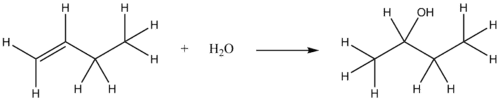Note that the first carbon in 1-butene started with two hydrogen atoms and the second carbon started with one hydrogen. Therefore, the hydrogen from water adds to the first carbon and the $$\ce{-OH}$$ group adds to the second carbon. When there are equal numbers of hydrogen atoms on both carbons in a double bond then the two products will form in approximately equal amounts. For example, the hydration of 2-pentene results in two products. In the first product, the $$\ce{-OH}$$ group is on the third carbon and in the second product, the $$\ce{-OH}$$ group is on the second carbon. While these two molecules will have similar properties, there will be differences.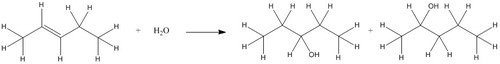## Condensation Reactions

A condensation reaction is a reaction in which two molecules combine to form a single molecule. A small molecule, often water, is usually removed during a condensation reaction. Amino acids are important biological molecules that have an amine functional group on one end of the molecule and a carboxylic acid functional group on the other end. When two amino acids combine in a condensation reaction, a covalent bond forms between the amine nitrogen of one amino acid and the carboxyl carbon of the second amino acid. A molecule of water is then removed as a second product (see figure below).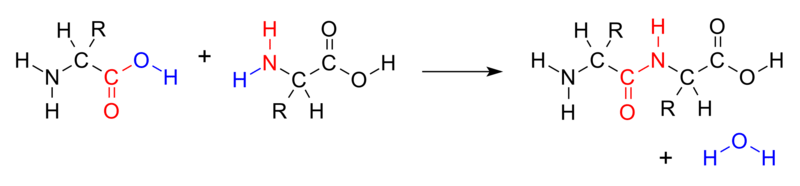Figure $$\PageIndex{1}$$: Amino acids join together to form a molecule called a dipeptide. The $$\ce{-OH}$$ from the carboxyl group of one amino acid combines with a hydrogen atom from the amine group of the other amino group to produce water (blue).

This reaction forms a molecule called a dipeptide, and the resulting carbon-nitrogen covalent bond is often called a peptide bond. When repeated numerous times, a long molecule called a protein is eventually produced.

An esterification is a condensation reaction in which an ester is formed from an alcohol and a carboxylic acid. Esterification is a subcategory of condensation reactions because a water molecule is produced in the reaction. The reaction can be catalyzed by a strong acid, usually sulfuric acid. When the carboxylic acid, butanoic acid, is heated with an excess of methanol and a few drops of sulfuric acid, the ester methyl butanoate is produced. Methyl butanoate has the scent of pineapples. The reaction is shown below with both molecular and structural formulas. Esterification reactions are reversible.

$\ce{CH_3CH_2CH_2COOH} + \ce{HOCH_3} \overset{\ce{H_2SO_4}}{\rightarrow} \ce{CH_3CH_2CH_2COOCH_3} + \ce{H_2O}$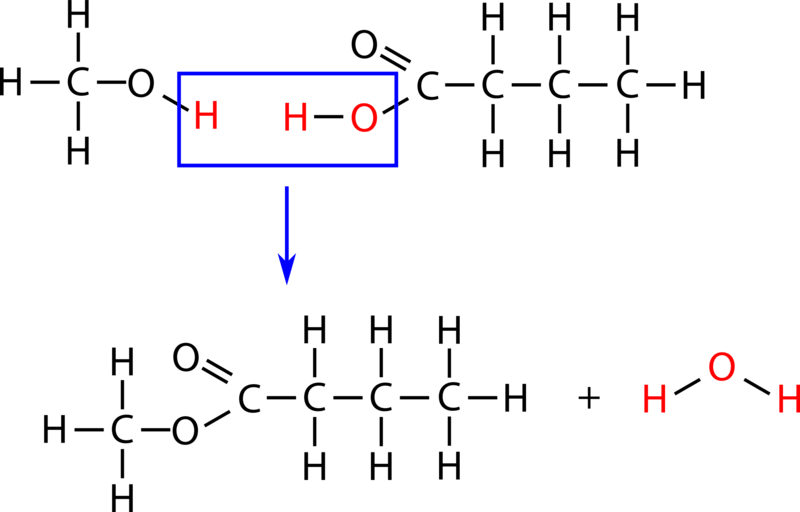Saponification describes the alkaline hydrolysis reaction of an ester. The term saponification originally described the hydrolysis of long-chain esters called fatty acid esters to produce soap molecules, which are the salts of fatty acids. One such soap molecule is sodium stearate, formed from the hydrolysis of ethyl stearate.

$\begin{array}{ccccccc} \ce{C_{17}H_{35}COOC_2H_5} & + & \ce{NaOH} & \rightarrow & \ce{C_{17}H_{35}COO^-Na^+} & + & \ce{C_2H_5OH} \\ \text{ethyl stearate} & & & & \text{sodium stearate (soap)} & & \end{array}$

## Hydrolysis Reactions

Hydrolysis is the reverse of condensation. "Hydro" indicates that water is involved and "lysis" means to break apart. In a hydrolysis reaction, water is added and the molecule breaks apart, usually at a $$\ce{C-O-C}$$ linkage.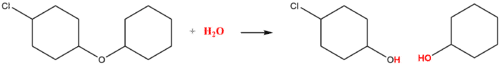## Polymerization

Polymers are very different than the other kinds of organic molecules that you have seen so far. Whereas other compounds are of relatively low molar mass, polymers are giant molecules of very high molar mass. Polymers are the primary components of all sorts of plastics and related compounds. A polymer is a large molecule formed of many smaller molecules covalently bonded to one another in a repeating pattern. The small molecules that make up the polymer are called monomers. Polymers are generally formed by either addition or condensation reactions. Teflon (see figure below) is a non-reactive, non-stick coating used on cookware as well as in containers and pipes for reactive or corrosive chemicals.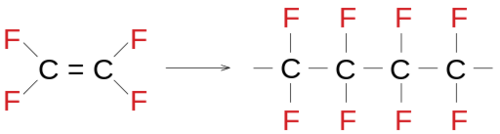Figure $$\PageIndex{2}$$: Polytetrafluoroethylene (also known as Teflon) is formed from the polymerization of tetrafluoroethylene.